Next: 2.2 Transport Kinetics Up: 2. Process Modeling Previous: 2. Process Modeling

# 2.1 Continuum Approach

Since the aim of semiconductor processing is the modification of the wafer surface, an appropriate model to describe the solid body is required. The natural way is an atomistic description, where the process is simulated using cellular automata or Monte Carlo techniques [13,33,47,78,118]. These atomistic approaches are able to predict characteristics as a microstructure or as surface roughness. However, the atomistic treatment is computationally very intensive and is therefore limited to small structures.

If the main concern is the prediction of the final shape of the structure, and not the microscopic properties, and if the typical structure sizes are much larger than the typical lattice constant, the solid body can be described as a continuum. The continuum approach mathematically represents the solid body as a region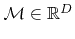with the surface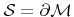as its boundary. Here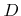is the number of dimensions. Material deposition or removal is simply described by moving the interfacewith respect to the deposition or removal rates on the surface.

Subsequent process steps often result in a multi-layer structure consisting of different material types. A common assumption within the continuum approach is that process relevant properties are homogeneous within each material region. Furthermore, the transition between two material layers is assumed to be abrupt. Hence, the entire geometry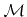can be described by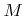disjoint material regions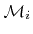which fulfill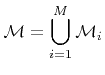and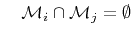for all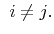(2.1)

As a consequence, knowing the surface and the interfaces between the different material regions is sufficient to describe the different material regions.Next: 2.2 Transport Kinetics Up: 2. Process Modeling Previous: 2. Process Modeling

Otmar Ertl: Numerical Methods for Topography Simulation